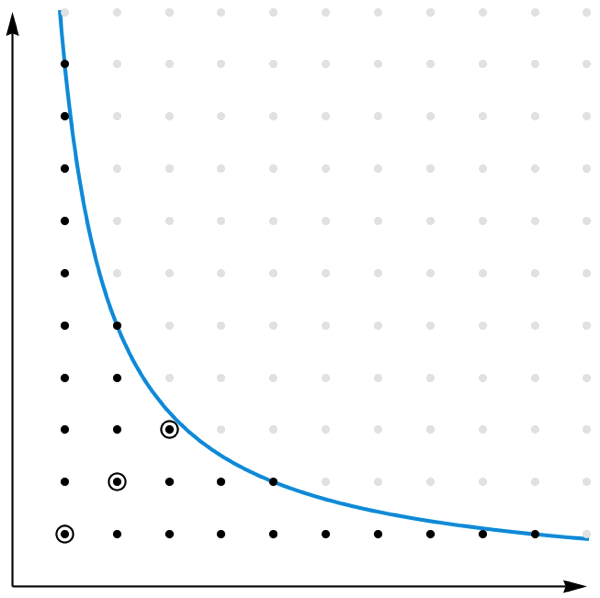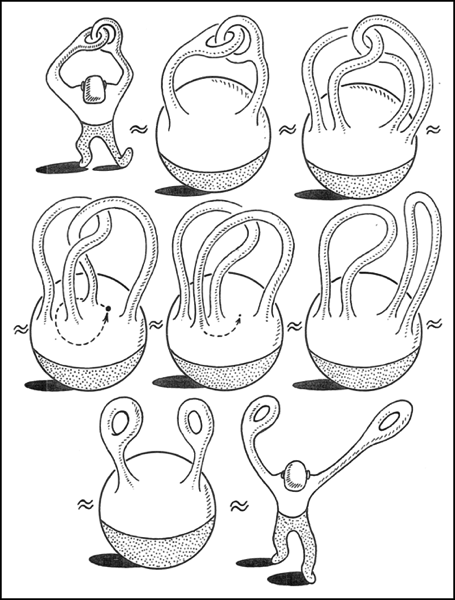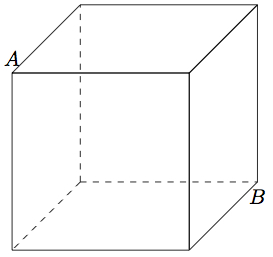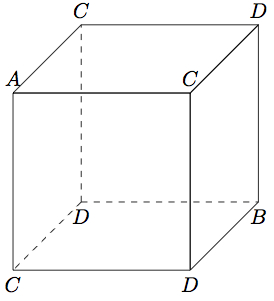# Letter 01 to IAS2014

50 分以上：2 人
40–49：3 人
30–39：10 人
20–29：15 人
10–19：11 人
0–9： 9 人

##### 第一题$\lambda y = a x \qquad \qquad y - a = -2 \lambda a x$$y (y-a) = -2 a^2 x^2$$\frac{x^2}{1/8} + \frac{(y-a/2)^2}{(a/2)^2} = 1$

•$a = \frac{1}{\sqrt{2}}$ 时，方程（1）是圆方程，故不存在合乎题意的定点$E$$F$
•$0 < a < \frac{1}{\sqrt{2}}$ 时，方程（1）表示椭圆，焦点$E\ \left( \frac{1}{2} \sqrt{\frac{1}{2}-a}, \frac{a}{2} \right)$$F\ \left( -\frac{1}{2} \sqrt{\frac{1}{2}-a}, \frac{a}{2} \right)$ 为合乎题意的两个定点。
•$\frac{1}{\sqrt{2}} < a$ 时，方程（1）表示椭圆，焦点$E\ \left( 0, \frac{1}{2} \left(a + \sqrt{\frac{1}{2}-a} \right) \right)$$F\ \left(0, \frac{1}{2} \left(a - \sqrt{\frac{1}{2}-a} \right) \right)$ 为合乎题意的两个定点。
##### 第二题$f(n) = \left[ \frac{n}{1} \right] + \left[ \frac{n}{2} \right] + \cdots + \left[ \frac{n}{n} \right] + \left[ \sqrt{n} \right]$ 容易得到$f(1) = 2$，这是一个偶数。接下来我们只要证明，对于所有的正整数$k$$f(k+1) - f(k)$ 的结果都是一个偶数。注意，如果$i$ 正好是$k+1$ 的一个约数，那么$[(k+1)/i] - [k/i]$ 将会等于1，否则$[(k+1)/i] - [k/i]$ 都会等于$0$。于是我们有$f(k+1) - f(k) = \sigma(k+1) + [\sqrt{k+1}] - [\sqrt{k}]$ 其中$\sigma(k+1)$ 表示$k+1$ 的约数的个数。##### 第三题$P( HIV \mid +) = \frac{ P(HIV) }{ P(+) } P( + \mid HIV) = \frac{ P(HIV) \cdot P(+ \mid HIV)}{P(HIV) \cdot P(+ \mid HIV) + P(-HIV) \cdot P(+ \mid -HIV)}$

##### 第四题##### 第五题$x = 1 + y \qquad y = 1 + \frac{1}{3}x + \frac{2}{3}z \qquad z = 1 + \frac{2}{3}y$

##### 第六题$u_i(v_i,b_i,p_i) = \begin{cases} v_i - p_i, & b_i > b_1,b_2,\ldots,b_{i-1}, \quad b_i \ge b_{i+1},\ldots,b_{10} \\\ 0, & otherwise \end{cases}$

• 如果$p_i \ge v_i$，那么出价$d_i$ 只会有两种结果，一是赢得这个物品，这时他的利益为$v_i - p_i \le 0$，非正数；二是没有赢得这个物品，利益为 0。这时，如果第 i 个人出价$v_i$，他保证可以得到 0。
• 如果$p_i < v_i$，那么出价$v_i$ 保证第 i 个人赢得物品，支付$p_i$，利益是正数。这时，如果第 i 个人出价$d_i$，有可能$d_i$ 比较小，没赢得这个物品（利益 0），有可能赢得这个物品，但收益与出价$v_i$ 时一致。

##### 第七题

• 如果$A \in A$，于是根据$A$ 的定义，$A \not\in A$，矛盾。
• 如果$A \not\in A$，则满足集合$A$ 中元素需要满足的性质，于是$A \in A$，矛盾。

##### 成绩公布

SYD：20 = 10（$P$ 坐标计算正确，椭圆方程错误，基于错误的椭圆方程分三种情况算的$E$$F$ 两点）+ 0（空）+ 8（阳性概率错误，艾滋病 + 阳性的概率正确，使用贝叶斯公式正确）+ 0 + 2（三秒到达 $$B$$ 的概率正确）+ 0 + 0

smile：28 = 5（$P$ 坐标计算正确，知道可能是椭圆焦点）+ 0（空）+ 15 + 1（回答可以，图示无法理解）+ 4（三、五秒到达$B$ 的概率正确）+ 0 + 3（回答不是，全体集合的全体属于自己）

MODii：43 = 12（一种情况计算错误） + 7（使用数学归纳法、质数情况的讨论正确）+ 15 + 0 + 5（不回到$A$ 的情况讨论基本正确）+ 0 + 4（回答不是，全体集合的全体属于自己，回答 ZF 公理）

K：23 = 4（$P$ 坐标计算正确）+ 2（证明初始情况）+ 0 + 0 + 2（三秒到达$B$ 的概率正确）+ 0（空）+ 15

Cam：26 = 14（一种情况计算错误）+ 0（空）+ 0 + 0（空）+ 0（空）+ 9（回答正确，缺解释）+ 3（回答不是，全体集合的全体属于自己）

xtb：12 = 4（一种情况正确）+ 4（使用数学归纳法）+ 0 + 0 + 0 + 3（第五个买家的出价正确，缺解释）+ 1（回答不是）

SIM：35 = 2（直线方程正确）+ 0 + 15 + 0 + 15 + 0 + 3（回答不是，全体集合的全体属于自己）

[( )]：9 = 5（$P$ 坐标计算正确，知道可能是椭圆焦点）+ 4（使用数学归纳法）+ 0 + 0 + 0 + 0 + 0

YJ：8 = 4（$P$ 坐标计算正确）+ 2（证明初始情况）+ 0 + 0 + 0 + 2（三秒到达$B$ 的概率正确）+ 0 + 0

MH：26 = 5（$P$ 坐标计算正确，知道可能是椭圆焦点）+ 1（部分证明初始情况）+ 15 + 1（回答能）+ 0 + 3（第五、九个买家的出价部分正确，缺解释）+ 1（回答不是）

GF：13 = 6（椭圆方程计算正确）+ 4（使用数学归纳法）+ 0 + 0 + 2（三秒到达$B$ 的概率正确）+ 0 + 1（回答不是）

zx：1 = 0 + 0 + 0 + 1（回答可以）+ 0 + 0 + 0

RM：6 = 5（$P$ 坐标计算正确，知道可能是椭圆焦点）+ 0 + 0 + 0 + 0 + 1（第一个买家的出价部分正确）+ 0

Jack：24 = 6（$P$ 坐标计算正确，一种情况部分正确）+ 0 + 15 + 0 + 0 + 3（第五个买家的出价正确，缺解释）+ 0（空）

Z：18 = 1（一条直线方程正确）+ 0（空）+ 15 + 1（回答能）+ 0 + 0 + 1（回答不是）

olando：29 = 5（$P$ 坐标计算正确，知道可能是椭圆焦点）+ 0（空）+ 15 + 0 + 4（三、五秒到达$B$ 的概率正确）+ 2（第五个买家的出价部分正确）+ 3（回答不是，全体集合的全体属于自己）

xx：1 = 0 + 0 + 0 + 0 + 0 + 0 + 1（回答不是）

## One thought on “Letter 01 to IAS2014”

1. 五更听风

看到最后一题我想起维特根斯坦，又去重新翻了一遍逻辑哲学论

The reason why a function cannot be its own argument is that the sign for a function already contains the prototype of its argument, and it cannot contain itself. For let us suppose that the function F(fx) could be its own argument: in that case there would be a proposition 'F(F(fx))', in which the outer function F and the inner function F must have different meanings, since the inner one has the form O(f(x)) and the outer one has the form Y(O(fx)). Only the letter 'F' is common to the two functions, but the letter by itself signifies nothing. This immediately becomes clear if instead of 'F(Fu)' we write '(do) : F(Ou) . Ou = Fu'. That disposes of Russell's paradox. （3.333）

一个命题永远不能阐述自己，理性永远无法解释自身。

This site uses Akismet to reduce spam. Learn how your comment data is processed.